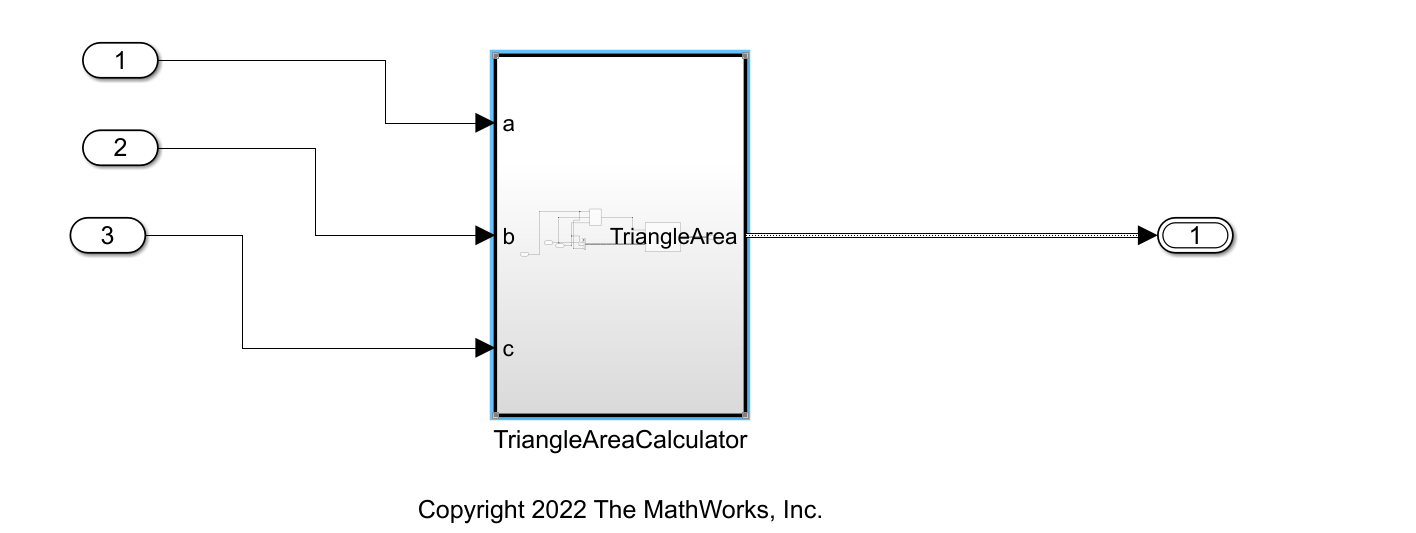# Generate Structured Text Code with VAR_IN_OUT Variables

This example shows how to model and generate structured text code that has `VAR_IN_OUT` variables. A `VAR_IN_OUT` variable is an input and output variable that is a part of the function block interface. Use `VAR_IN_OUT` variables to pass values to the function block by using the pass by reference method.

### Open the Model

`open_system('GenerateVarInOutVariables.slx')`The model consists of a subsystem `TriangleAreaCalculator` at the top level that accepts three inputs `a`, `b`, and `c`. The subsystem contains :

• A subsystem block `CalculateTriangleSemiPerimeter` that accepts `a`, `b`, and `c` as an input and generates an output` `s.

• A MATLAB® Function block `CalculateTriangleArea` that accepts `TriangleArea` and `s` as inputs and generates `TriangleArea` as the output.

### Model to Generate VAR_IN_OUT Variables

Generate `VAR_IN_OUT` variables by using a MATLAB Function block and declaring a function of type:

```function x = function_name(x) % body of function ```

Simulink® PLC Coder supports `VAR_IN_OUT `generation only from MATLAB Function blocks. This is the structure of the MATLAB Function Block:

```function TriangleArea = fcn(s,TriangleArea) intermediate_area = (s-TriangleArea.a)*(s-TriangleArea.b)*(s-TriangleArea.c); TriangleArea.area = sqrt(s*intermediate_area); ```

### Generate Structured Text Code

To generate structured text code,

1. In the Apps tab, click PLC Coder.

2. In the PLC Coder tab, clock Settings > PLC Code Generation Settings. Change the Target IDE to `3S CodeSys 2.3`. Click OK.

3. Select the subsystem block. In the PLC Code tab, click Generate PLC Code.

Alternatively, to generate structured text code from the MATLAB command line, use the `plcgeneratecode` function.

`generatedfiles = plcgeneratecode('GenerateVarInOutVariables/TriangleAreaCalculator');`
```### Generating PLC code for 'GenerateVarInOutVariables/TriangleAreaCalculator'. ### Using model settings from 'GenerateVarInOutVariables' for PLC code generation parameters. ### Begin code generation for IDE codesys23. ### Emit PLC code to file. ### Creating PLC code generation report GenerateVarInOutVariables_codegen_rpt.html. ### PLC code generation successful for 'GenerateVarInOutVariables/TriangleAreaCalculator'. ### Generated files: plcsrc/GenerateVarInOutVariables.exp ```

` `The generated structured text code contains `VAR_IN_OUT` variable declarations. The MATLAB Function block takes the variable `TriangleArea` as an input and generates the same variable as the output.

```FUNCTION_BLOCK TriangleAreaCalculator VAR_INPUT a: LREAL; b: LREAL; c: LREAL; END_VAR VAR_OUTPUT TriangleArea_e: TriangleArea; END_VAR VAR i0_CalculateTriangleArea: CalculateTriangleArea; END_VAR (* Outputs for Atomic SubSystem: '<Root>/TriangleAreaCalculator' *) (* BusCreator: '<S1>/Bus Creator' incorporates: * MATLAB Function: '<S1>/CalculateTriangleArea' * Outport: '<Root>/TriangleArea' *) TriangleArea_e.area := 0.0; TriangleArea_e.a := a; TriangleArea_e.b := b; TriangleArea_e.c := c; (* Product: '<S3>/Divide' incorporates: * Constant: '<S3>/Constant' * Outport: '<Root>/TriangleArea' * Sum: '<S3>/Add' *) i0_CalculateTriangleArea(b_s := ((a + b) + c) / 2.0, TriangleArea_i := TriangleArea_e); (* End of Outputs for SubSystem: '<Root>/TriangleAreaCalculator' *) END_FUNCTION_BLOCK FUNCTION_BLOCK CalculateTriangleArea VAR_INPUT b_s: LREAL; END_VAR VAR_IN_OUT TriangleArea_i: TriangleArea; END_VAR (* MATLAB Function 'TriangleAreaCalculator/CalculateTriangleArea': '<S2>:1' *) (* '<S2>:1:2' intermediate_area = (s-TriangleArea.a)*(s-TriangleArea.b)*(s-TriangleArea.c); *) (* '<S2>:1:3' TriangleArea.area = sqrt(s*intermediate_area); *) TriangleArea_i.area := SQRT((((b_s - TriangleArea_i.a) * (b_s - TriangleArea_i.b)) * (b_s - TriangleArea_i.c)) * b_s); END_FUNCTION_BLOCK TYPE TriangleArea: STRUCT area: LREAL; b: LREAL; c: LREAL; a: LREAL; END_STRUCT END_TYPE ```